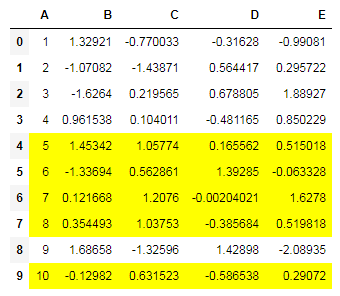﻿ Pandas styling: Write a Pandas program to highlight the entire row in Yellow where a specific column value is greater than 0.5 - w3resource

# Pandas styling Exercises: Write a Pandas program to highlight the entire row in Yellow where a specific column value is greater than 0.5

## Pandas styling: Exercise-10 with Solution

Create a dataframe of ten rows, four columns with random values. Write a Pandas program to highlight the entire row in Yellow where a specific column value is greater than 0.5.

Sample Solution :

Python Code :

``````import pandas as pd
import numpy as np
np.random.seed(24)
df = pd.DataFrame({'A': np.linspace(1, 10, 10)})
df = pd.concat([df, pd.DataFrame(np.random.randn(10, 4), columns=list('BCDE'))],
axis=1)
print("Original array:")
print(df)
print("\nDataframe - Highlight an entire row on specific condition:")

def highlight_greaterthan(x):
if x.C > .5:
return ['background-color: yellow']*5
else:
return ['background-color: white']*5
df.style.apply(highlight_greaterthan, axis=1)
``````

Original array:

```Original array:
A         B         C         D         E
0   1.0  1.329212       NaN -0.316280 -0.990810
1   2.0 -1.070816 -1.438713  0.564417  0.295722
2   3.0 -1.626404  0.219565  0.678805  1.889273
3   4.0  0.961538  0.104011       NaN  0.850229
4   5.0       NaN  1.057737  0.165562  0.515018
5   6.0 -1.336936  0.562861  1.392855 -0.063328
6   7.0  0.121668  1.207603 -0.002040  1.627796
7   8.0  0.354493  1.037528 -0.385684  0.519818
8   9.0  1.686583 -1.325963  1.428984 -2.089354
9  10.0 -0.129820  0.631523 -0.586538       NaN

Dataframe - Highlight an entire row on specific condition:
```

Sample Output:

``````